Miscellaneous

Chapter 7 Class 12 Integrals
Serial order wise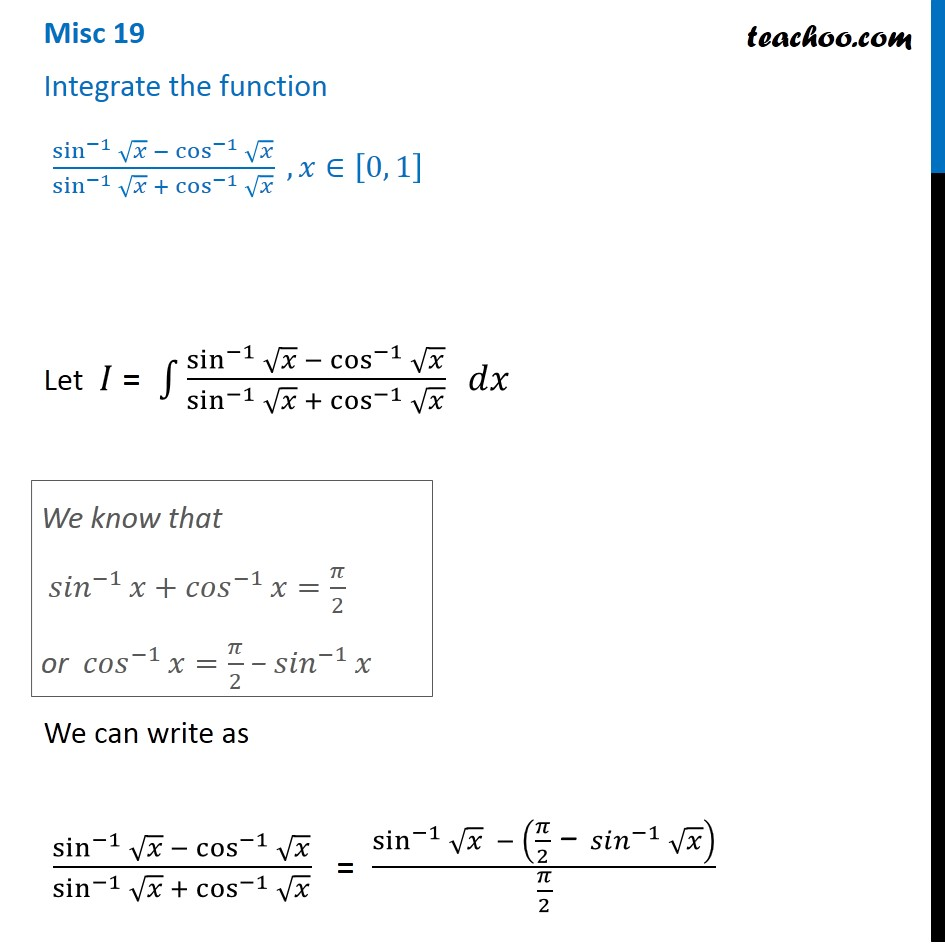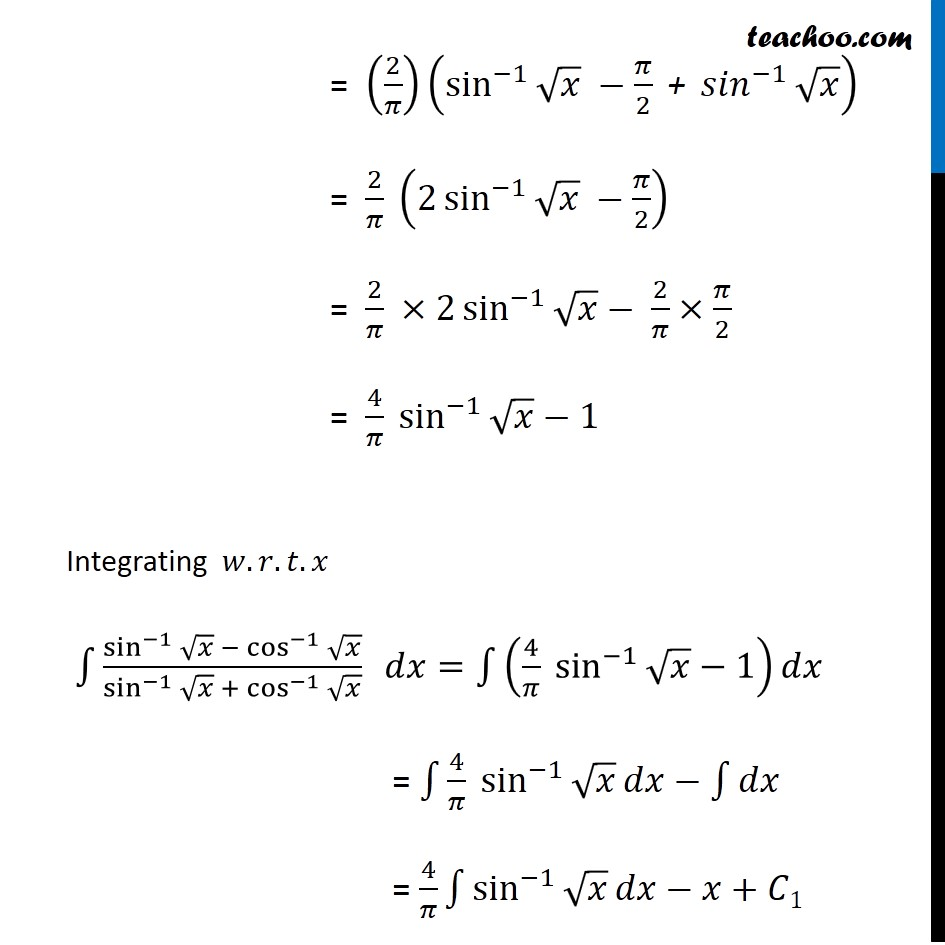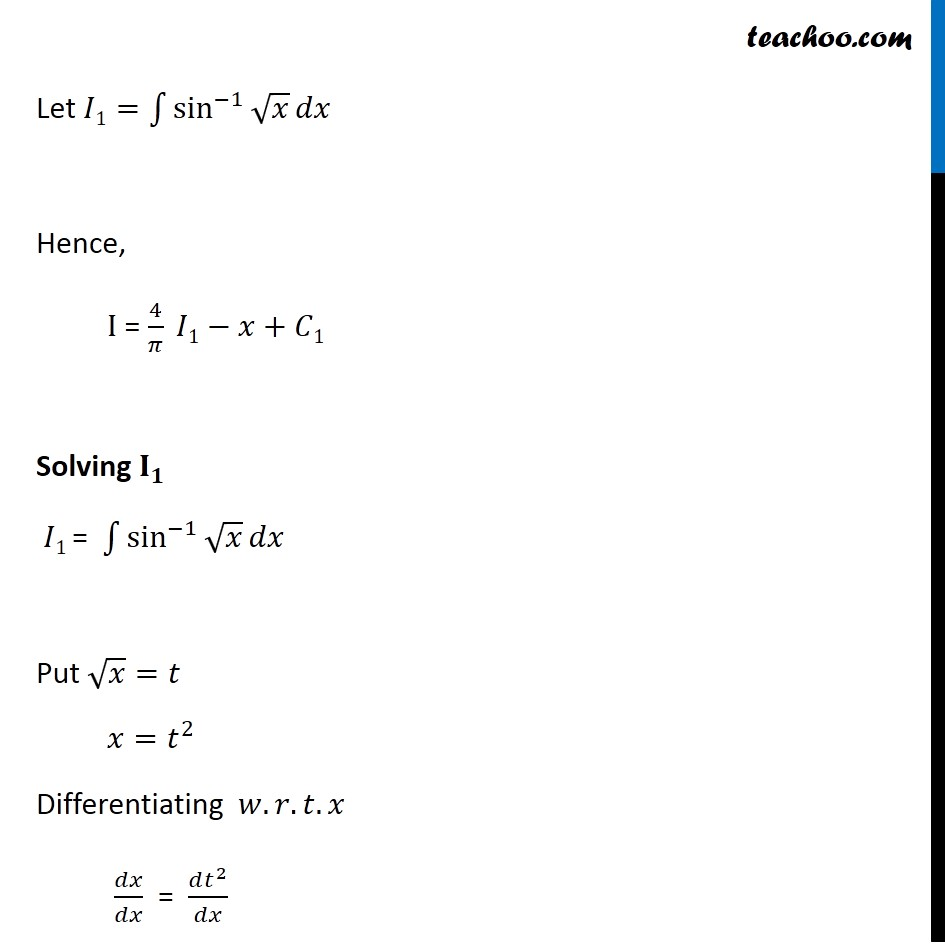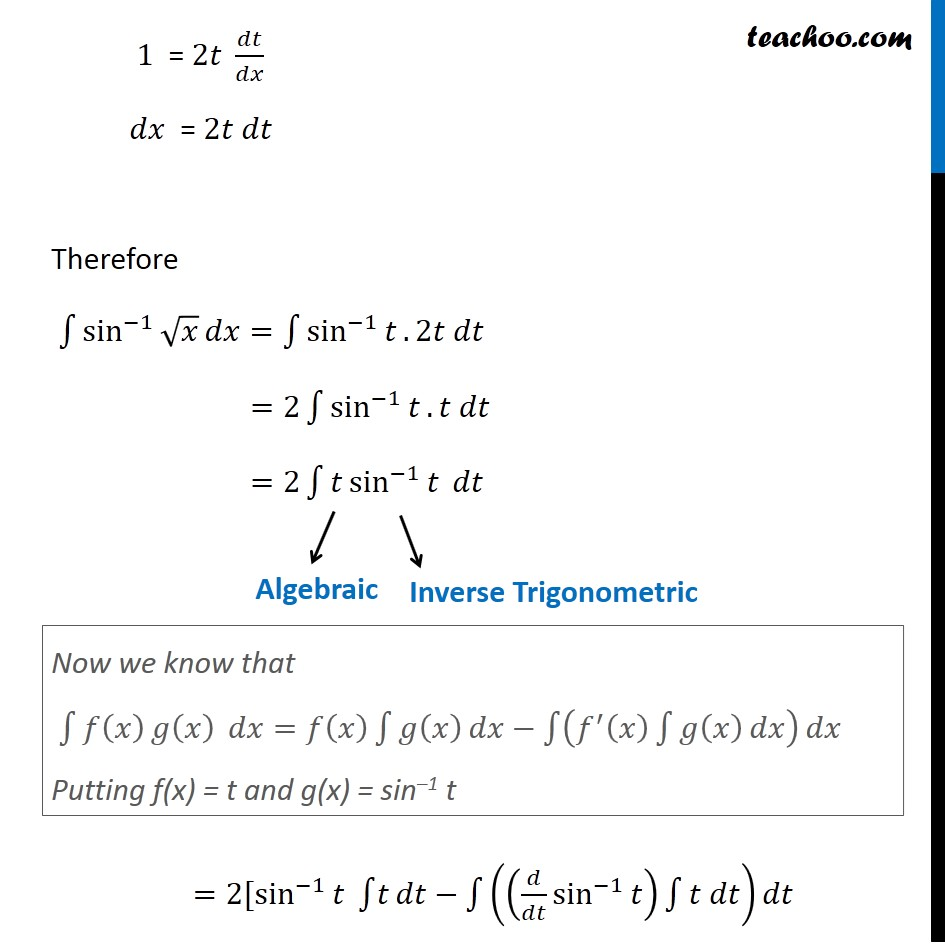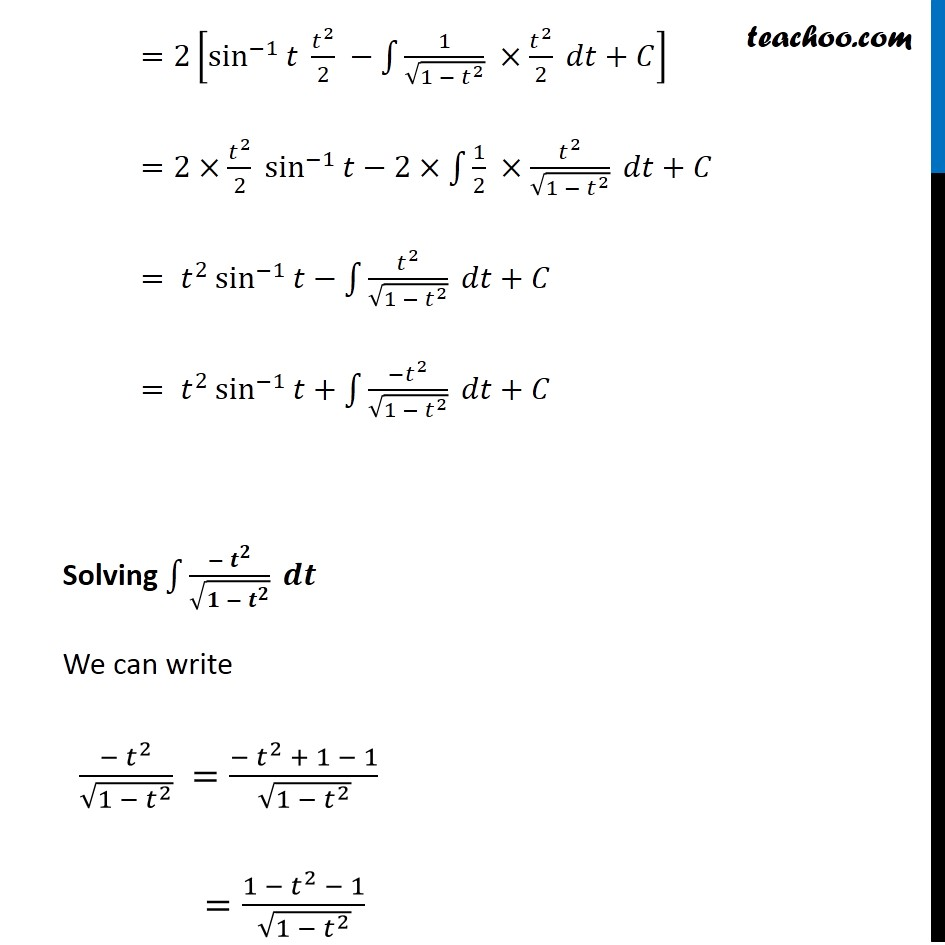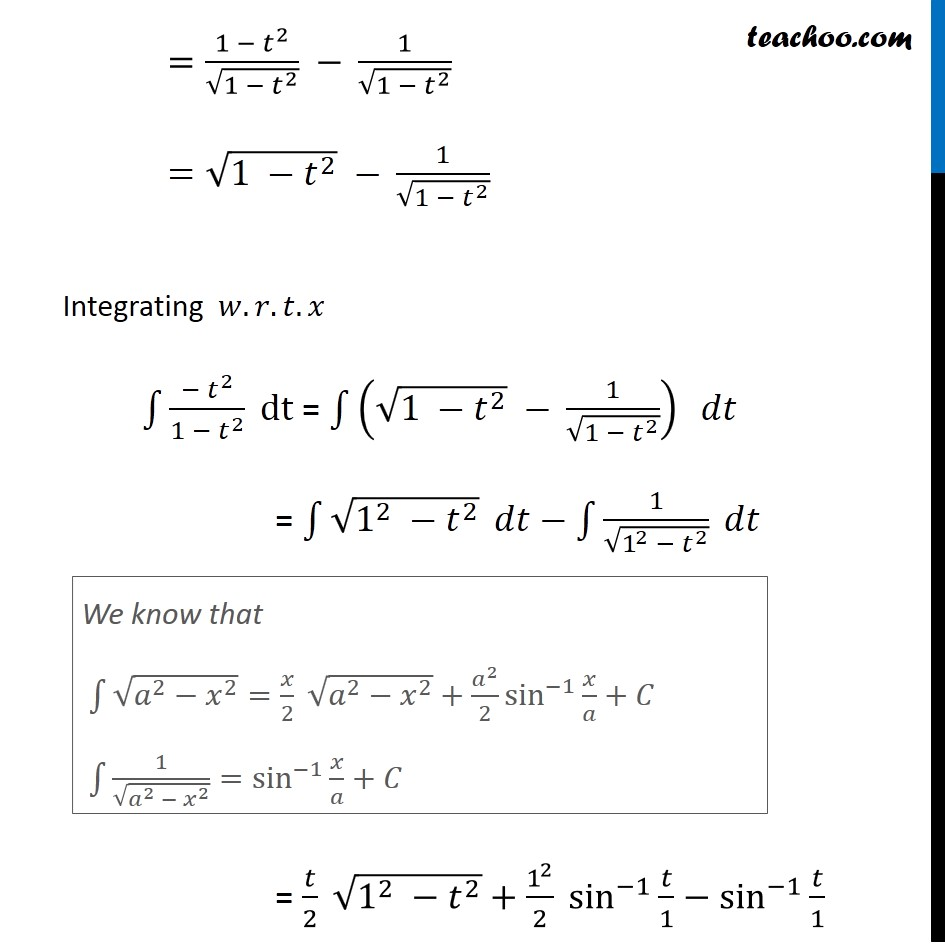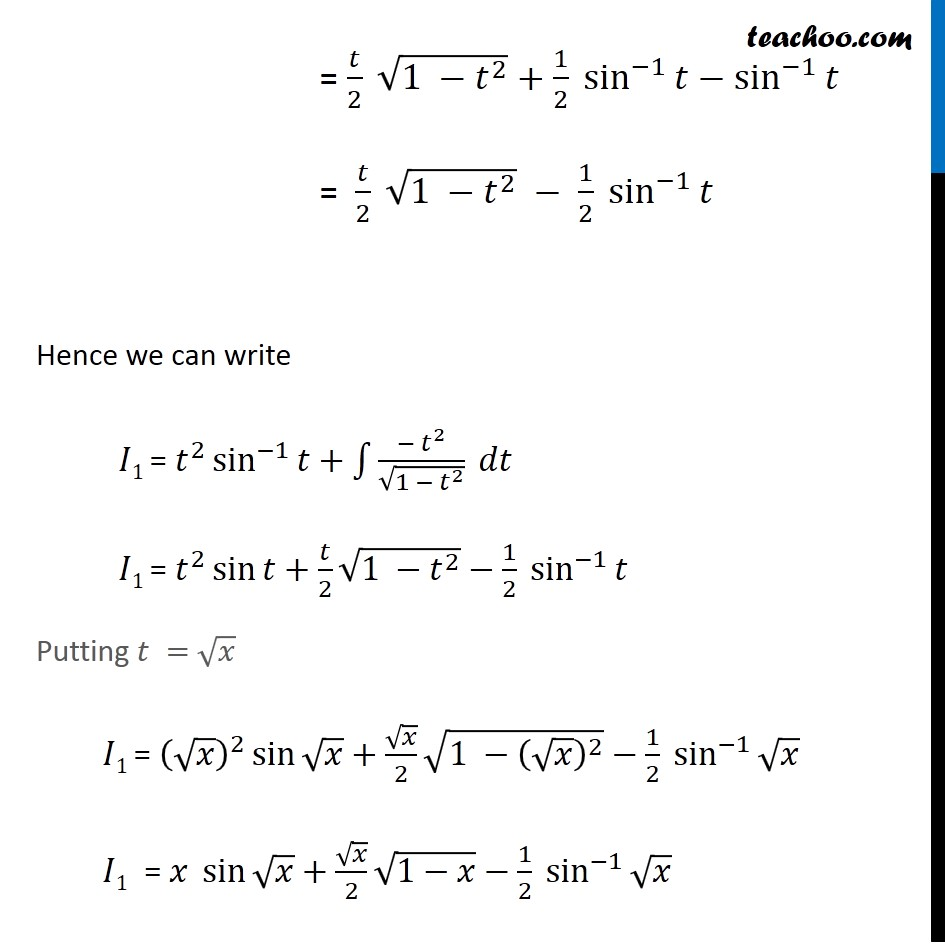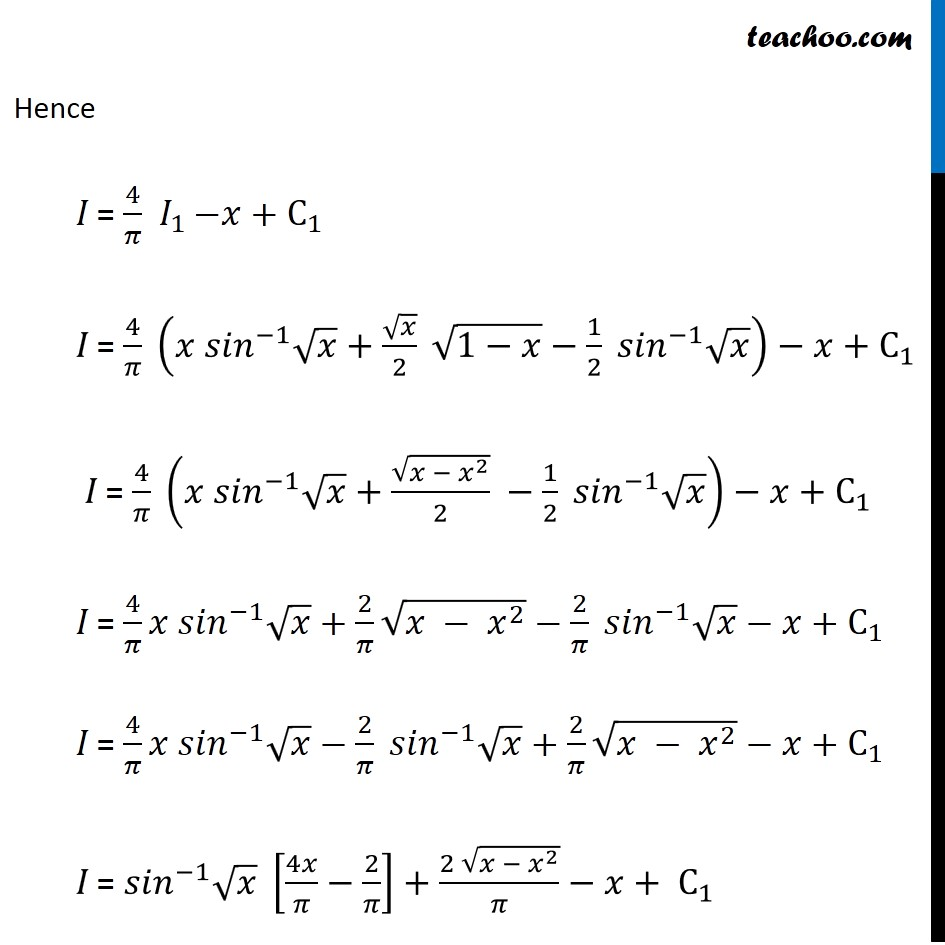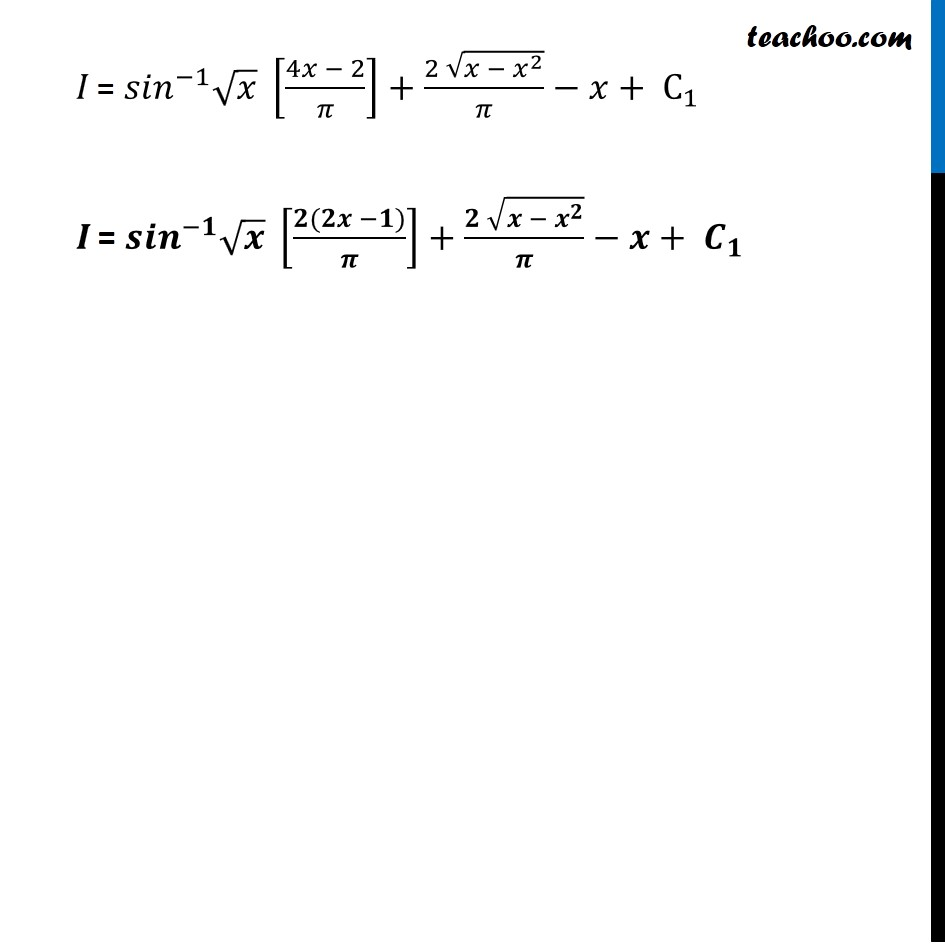Learn in your speed, with individual attention - Teachoo Maths 1-on-1 Class

### Transcript

Question 1 Integrate the function (sin^(−1)⁡√𝑥 − cos^(−1)⁡√𝑥)/(sin^(−1)⁡√𝑥 + cos^(−1)⁡√𝑥 ) , 𝑥∈[0, 1] Let 𝐼 = ∫1▒(sin^(−1)⁡√𝑥 − cos^(−1)⁡√𝑥)/(sin^(−1)⁡√𝑥 + cos^(−1)⁡√𝑥 ) 𝑑𝑥 We can write as (sin^(−1)⁡√𝑥 − cos^(−1)⁡√𝑥)/(sin^(−1)⁡√𝑥 + cos^(−1)⁡√𝑥 ) = (sin^(−1)⁡√𝑥 − (𝜋/2 " −" 〖 𝑠𝑖𝑛〗^(−1)⁡√𝑥 ))/(𝜋/2) We know that 〖𝑠𝑖𝑛〗^(−1)⁡𝑥+〖𝑐𝑜𝑠〗^(−1)⁡𝑥=𝜋/2 or 〖𝑐𝑜𝑠〗^(−1)⁡𝑥=𝜋/2 −〖 𝑠𝑖𝑛〗^(−1)⁡𝑥 = (2/𝜋)(sin^(−1)⁡√𝑥 −𝜋/2 " +" 〖 𝑠𝑖𝑛〗^(−1)⁡√𝑥 ) = 2/𝜋 (2 sin^(−1)⁡√𝑥 −𝜋/2) = 2/𝜋 ×2 sin^(−1)⁡√𝑥− 2/𝜋×𝜋/2 = 4/𝜋 sin^(−1)⁡√𝑥−1 Integrating 𝑤.𝑟.𝑡.𝑥 ∫1▒(sin^(−1)⁡√𝑥 − cos^(−1)⁡√𝑥)/(sin^(−1)⁡√𝑥 + cos^(−1)⁡√𝑥 ) 𝑑𝑥=∫1▒(4/𝜋 sin^(−1)⁡√𝑥−1) 𝑑𝑥 = ∫1▒〖4/𝜋 sin^(−1)⁡√𝑥 〗 𝑑𝑥−∫1▒𝑑𝑥 = 4/𝜋 ∫1▒sin^(−1)⁡√𝑥 𝑑𝑥−𝑥+𝐶1 Let 𝐼1=∫1▒sin^(−1)⁡√𝑥 𝑑𝑥 Hence, I = 4/𝜋 𝐼1−𝑥+𝐶1 Solving 𝐈_𝟏 𝐼1 = ∫1▒sin^(−1)⁡√𝑥 𝑑𝑥 Put √𝑥=𝑡 𝑥=𝑡^2 Differentiating 𝑤.𝑟.𝑡.𝑥 𝑑𝑥/𝑑𝑥 = (𝑑𝑡^2)/𝑑𝑥 1 = 2𝑡 𝑑𝑡/𝑑𝑥 𝑑𝑥 = 2𝑡 𝑑𝑡 Therefore ∫1▒sin^(−1)⁡√𝑥 𝑑𝑥=∫1▒sin^(−1)⁡𝑡 .2𝑡 𝑑𝑡 =2∫1▒sin^(−1)⁡𝑡 .𝑡 𝑑𝑡 =2∫1▒〖𝑡 sin^(−1)⁡〖𝑡 〗 〗 𝑑𝑡 =2[sin^(−1)⁡〖𝑡 〗 ∫1▒𝑡 𝑑𝑡−∫1▒((𝑑/𝑑𝑡 sin^(−1)⁡𝑡 ) ∫1▒〖𝑡 𝑑𝑡〗) 𝑑𝑡 Now we know that ∫1▒〖𝑓(𝑥) 𝑔⁡(𝑥) 〗 𝑑𝑥=𝑓(𝑥) ∫1▒𝑔(𝑥) 𝑑𝑥−∫1▒(𝑓^′ (𝑥) ∫1▒𝑔(𝑥) 𝑑𝑥) 𝑑𝑥 Putting f(x) = t and g(x) = sin–1 t =2[sin^(−1)⁡〖𝑡 〗 𝑡^2/2 −∫1▒1/√(1 −〖 𝑡〗^2 ) ×𝑡^2/2 𝑑𝑡+𝐶] =2×𝑡^2/2 〖 sin^(−1)〗⁡𝑡−2×∫1▒〖1/2 ×𝑡^2/√(1 −〖 𝑡〗^2 )〗 𝑑𝑡+𝐶 = 𝑡^2 sin^(−1)⁡𝑡−∫1▒𝑡^2/√(1 −〖 𝑡〗^2 ) 𝑑𝑡+𝐶 = 𝑡^2 sin^(−1)⁡𝑡+∫1▒(−𝑡^2)/√(1 −〖 𝑡〗^2 ) 𝑑𝑡+𝐶 Solving ∫1▒〖− 𝒕〗^𝟐/√(𝟏 −〖 𝒕〗^𝟐 ) 𝒅𝒕 We can write (− 𝑡^2)/√(1 −〖 𝑡〗^2 ) =(〖− 𝑡〗^2 + 1 − 1)/√(1 −〖 𝑡〗^2 ) =(〖1 − 𝑡〗^2 − 1)/√(1 −〖 𝑡〗^2 ) =〖1 − 𝑡〗^2/√(1 −〖 𝑡〗^2 ) −" " 1/√(1 −〖 𝑡〗^2 ) =√(1 −〖 𝑡〗^2 ) −" " 1/√(1 −〖 𝑡〗^2 ) Integrating 𝑤.𝑟.𝑡.𝑥 ∫1▒(− 𝑡^2)/(1 −〖 𝑡〗^2 ) dt = ∫1▒〖(√(1 −〖 𝑡〗^2 ) −" " 1/√(1 −〖 𝑡〗^2 )) 〗 𝑑𝑡 = ∫1▒√(1^2 −〖 𝑡〗^2 ) 𝑑𝑡−∫1▒1/√(1^2 −〖 𝑡〗^2 ) 𝑑𝑡 = 𝑡/2 √(1^2 −〖 𝑡〗^2 )+1^2/2 sin^(−1)⁡〖𝑡/1〗−sin^(−1)⁡〖𝑡/1〗 We know that ∫1▒√(𝑎^2−𝑥^2 )=𝑥/2 √(𝑎^2−𝑥^2 )+𝑎^2/2 sin^(−1)⁡〖𝑥/𝑎〗+𝐶 ∫1▒1/√(𝑎^2 − 𝑥^2 )=sin^(−1)⁡〖𝑥/𝑎〗+𝐶 = 𝑡/2 √(1 −〖 𝑡〗^2 )+1/2 sin^(−1)⁡𝑡−sin^(−1)⁡𝑡 = 𝑡/2 √(1 −〖 𝑡〗^2 ) − 1/2 sin^(−1)⁡𝑡 Hence we can write 𝐼1 = 𝑡^2 sin^(−1)⁡𝑡+∫1▒(− 𝑡^2)/√(1 −〖 𝑡〗^2 ) 𝑑𝑡 𝐼1 = 𝑡^2 sin⁡𝑡+𝑡/2 √(1 −〖 𝑡〗^2 )−1/2 sin^(−1)⁡𝑡 Putting 𝑡 = √𝑥 𝐼1 = (√𝑥)^2 sin⁡√𝑥+√𝑥/2 √(1 −〖 (√𝑥)〗^2 )−1/2 sin^(−1)⁡√𝑥 𝐼1 = 𝑥 sin⁡√𝑥+√𝑥/2 √(1−𝑥)−1/2 sin^(−1)⁡√𝑥 Hence 𝐼 = 4/𝜋 〖 𝐼〗_(1 )−𝑥+C_1 𝐼 = 4/𝜋 (𝑥 〖𝑠𝑖𝑛〗^(−1) √𝑥+√𝑥/2 √(1−𝑥)−1/2 〖𝑠𝑖𝑛〗^(−1) √𝑥)−𝑥+C_1 𝐼 = 4/𝜋 (𝑥 〖𝑠𝑖𝑛〗^(−1) √𝑥+√(𝑥 − 𝑥^2 )/2 −1/2 〖𝑠𝑖𝑛〗^(−1) √𝑥)−𝑥+C_1 𝐼 = 4/𝜋 𝑥 〖𝑠𝑖𝑛〗^(−1) √𝑥+2/𝜋 √(𝑥 − 𝑥^2 )−2/𝜋 〖𝑠𝑖𝑛〗^(−1) √𝑥−𝑥+C_1 𝐼 = 4/𝜋 𝑥 〖𝑠𝑖𝑛〗^(−1) √𝑥−2/𝜋 〖𝑠𝑖𝑛〗^(−1) √𝑥+2/𝜋 √(𝑥 − 𝑥^2 )−𝑥+C_1 𝐼 = 〖𝑠𝑖𝑛〗^(−1) √𝑥 [4𝑥/𝜋−2/𝜋]+(2 √(𝑥 − 𝑥^2 ))/𝜋−𝑥+ C_1 𝐼 = 〖𝑠𝑖𝑛〗^(−1) √𝑥 [(4𝑥 − 2)/𝜋]+(2 √(𝑥 − 𝑥^2 ))/𝜋−𝑥+ C_1 𝑰 = 〖𝒔𝒊𝒏〗^(−𝟏) √𝒙 [(𝟐(𝟐𝒙 −𝟏))/𝝅]+(𝟐 √(𝒙 − 𝒙^𝟐 ))/𝝅−𝒙+ 𝑪_𝟏